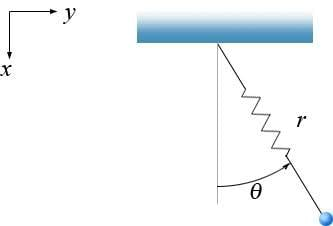# Spring pendulum with friction (Lagrange?)

## Homework Statement

Consider a pendulum with a spring as in the following diagram:The bob has a mass $m$. The spring has a spring constant $k$ and an unextended length $\ell$. We can not ignore air friction.

Assume the initial velocity and horizontal displacement are parallel, so that the motion of the pendulum is in the plane. It is not a 'spherical' pendulum.

I need to show that the equations of motion satisfy the following:
$$m\left( r'' - r (\theta ')^2 \right) + cr' + k(r-\ell) = mg \cos \theta$$
$$m \left( r \theta '' + 2 r' \theta ' \right) + cr \theta ' = - mg \sin \theta$$

## Homework Equations

The forces on the bob are the force of gravity, the spring force and the air friction:
$$\mathbf{F}_g = mg \mathbf{\hat{i}}$$
$$\mathbf{F}_s = -k \left(r - \ell \right) \mathbf{\hat{e_r}}$$
$$\mathbf{F}_f = -c \mathbf{r}'$$
(where c is a positive friction coefficient)

## The Attempt at a Solution

I can use the Lagrangian to find the equations of motion, but I don't have a clue what to do with the friction force..??

Using the Lagrangian, the kinetic energy T is:
$$T = \frac{1}{2} m v^2 = \frac{1}{2} m (x' ^2 + y'^2) = \frac{1}{2} m \left[ (r')^2 + r^2 (\theta ')^2\right]$$

The potential V is the sum of the gravitational potential V_g, the spring potential V_s, and...??? Frictional potential? I don't think I can write the frictional force as a potential, can I?
Anyway:
$$V = -mgr \cos \theta + \frac{1}{2}k(r-\ell)^2 + ...?$$

$$L = T - V = \frac{1}{2} m \left[ (r')^2 + r^2 (\theta ')^2\right] +mgr \cos \theta - \frac{1}{2}k(r-\ell)^2$$

For r, we have:
$$\frac{\partial}{\partial t}\left( \frac{\partial L}{\partial r'} \right) = \frac{\partial L}{\partial r}$$
From this I get:
$$m\left( r'' - r (\theta ')^2 \right) + k(r-\ell) = mg \cos \theta$$

For theta, we have:
$$\frac{\partial}{\partial t}\left( \frac{\partial L}{\partial \theta '} \right) = \frac{\partial L}{\partial \theta}$$
And I get:
$$m \left( r \theta '' + 2 r' \theta ' \right) = - mg \sin \theta$$

So as expected, I get the correct equations, without the frictional terms...

How can I calculate the frictional terms? Can I use the Lagrangian, or do I have to use normal force analysis? It doesn't make sense in my mind to use both the Lagrangian and force analysis... ?

Thanks!

## The Attempt at a Solution

In the case of dissipative forces, the Lagrangian is unchanged. However, the Euler-Lagrange equation changes.

The Euler-Lagrange equation is typically derived by extremizing the action:

$$\delta S = \delta\int{L(x, \dot x)} dt = 0$$

However, another equivalent starting point is the "[URL [Broken] principle[/URL], which states that the virtual work done by a system is 0.

The derivation from then on is relatively involved (Goldstein 3Ed pg16 - pg24), but one finds that if we assume that the force is conservative, we get the usual Euler-Lagrange equation. However, if we assume that the force has conservative and dissipative terms, we find out that the Euler-Lagrange equation equation needs to be modified by adding a dissipation function.

The results are summarized in this "www.phys.uri.edu/~gerhard/PHY520/mln9.pdf"[/URL].

In the end, what all this really means is just

$$\frac{d}{dt}\left(\frac{\partial L}{\partial \dot q_j}\right) - \frac{\partial L}{\partial q_j} = Q_j$$

, where $$Q_j$$ is the dissipative force. Which is probably not surprising at all.

Last edited by a moderator:
Thanks, got it!